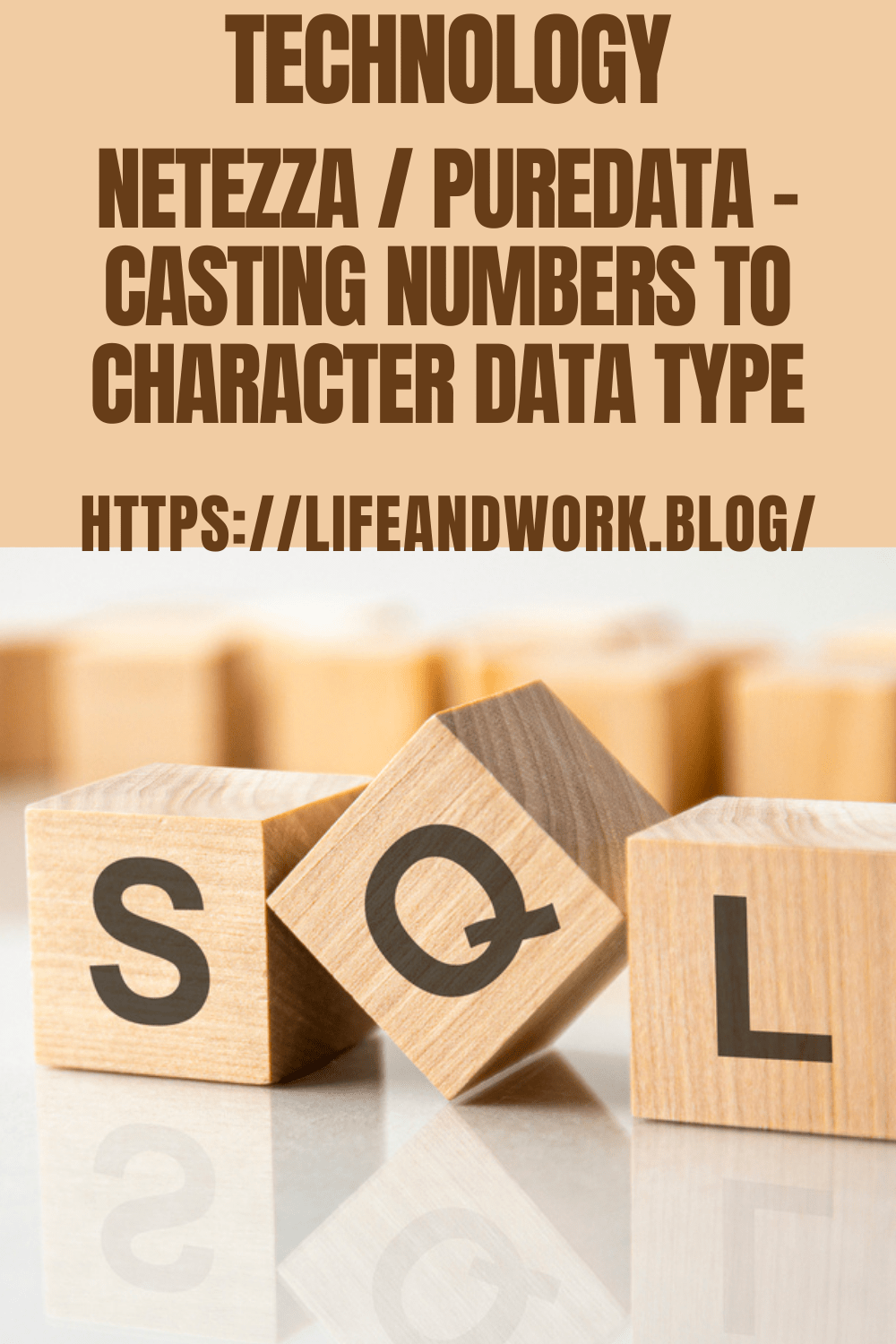# Netezza / PureData – Casting Numbers to Character Data TypeI noticed that someone has been searching for this example on my site, so, here is a quick example of how to cast number data to a character data type.  I ran this SQL example in Netezza and it worked fine.

# Basic Casting Conversion Format

cast(<<FieldName>> as <<IntegerType_or_Alias>>) as <<FieldName>>

# Example Casting Number to Character Data Type SQL

SELECT
—-Casting Integer to Character Data Type —————

SUBMITDATE_SRKY as  SUBMITDATE_SRKY_INTEGER
, cast(SUBMITDATE_SRKY as  char(10)) as Integer_to_CHAR
, cast(SUBMITDATE_SRKY as Varchar(10)) as Integer_to_VARCHAR
, cast(SUBMITDATE_SRKY as Varchar(10)) as Integer_to_VARCHAR
, cast(SUBMITDATE_SRKY as Nchar(10)) as Integer_to_NCHAR
, cast(SUBMITDATE_SRKY as NVarchar(10)) as Integer_to_NVARCHAR

—-Casting Double Precision Number to Character Data Type —————

, HOST_CPU_SECS as DOUBLE_PRECISION_NUMBER
, cast(HOST_CPU_SECS as  char(30)) as DOUBLE_PRECISION_to_CHAR
, cast(HOST_CPU_SECS as Varchar(30)) as DOUBLE_PRECISION_to_VARCHAR
, cast(HOST_CPU_SECS as Varchar(30)) as DOUBLE_PRECISION_to_VARCHAR
, cast(HOST_CPU_SECS as Nchar(30)) as DOUBLE_PRECISION_to_NCHAR
, cast(HOST_CPU_SECS as NVarchar(30)) as DOUBLE_PRECISION_to_NVARCHAR

—-Casting Numeric to Character Data Type —————

, TOTALRUNTIME  as NUMERIC_FIELD
, cast(TOTALRUNTIME as  char(30)) as NUMERIC_FIELD_to_CHAR
, cast(TOTALRUNTIME as Varchar(30)) as NUMERIC_FIELD_to_VARCHAR
, cast(TOTALRUNTIME as Varchar(30)) as NUMERIC_FIELD_to_VARCHAR
, cast(TOTALRUNTIME as Nchar(30)) as NUMERIC_FIELD_to_NCHAR
, cast(TOTALRUNTIME as NVarchar(30)) as NUMERIC_FIELD_to_NVARCHAR
FROM NETEZZA_QUERY_FACT ;